﻿ Stochastic Models for Forecasting Inflation Rate: Empirical Evidence from GreecePublications are Open
Access in this journal
Article Versions
Export Article
• Normal Style
• MLA Style
• APA Style
• Chicago Style
Research Article
Open Access Peer-reviewed

### Stochastic Models for Forecasting Inflation Rate: Empirical Evidence from Greece

Chaido Dritsaki , Leonidas Petrakis
Journal of Finance and Economics. 2017, 5(3), 145-155. DOI: 10.12691/jfe-5-3-7
Published online: June 13, 2017

### Abstract

The main aim of the macroeconomic policy of every country is to achieve a continuously high economic development combined with low inflation rates. A low, stable inflation level together with a sustainable budget deficit, a realistic exchange rate and a suitable real interest rate, consist indices of a stable macroeconomic environment. The diverse economic policies applied in Greece during the period under consideration, led to high inflation periods and guided the country to IMF since 2010. The high inflation rate in Greece was mainly generated by the increasing money supply. The present paper is an effort for the development of a stochastic model which will enable us to forecast inflation, taking into consideration the economic periods Greece went through. For this reason, we use the Box-Jenkins methodology by constructing a seasonal ARIMA model in order to represent the mean component using the past values. Then we incorporate a GARCH model to represent its volatility. The results of all tests reveal that the seasonal SARIMA(2,1,2)(0,1,1)12-EGARCH(1,1) model with the distribution of the generalized error (GED) and the Marquardt algorithm is the most suitable for forecasting the inflation in Greece. The forecasting results of this model showed that inflation in the following months will range from 0 to 1%.

### 1. Introduction

In economy, inflation is a stable increase of the price of goods and services. Therefore, inflation reflects the decrease of the purchasing power of citizens. One inflation measure is the annual percentage change of the general rate of prices which is referred as price rate of the consumer. Inflation can affect economies positively or negatively. The positive impacts of inflation include the decrease of the burden of public and private debt, with the central banks maintaining positive interest rates, but also the decrease of the unemployment due to the stiffness of the salaries. The negative consequences of inflation include the increase of opportunity cost, the retention of money and the discouragement of investments and money savings.

Many economists believe that the high rates of inflation are caused by the excessive increase of money offer 1. 2 supports that under the circumstances of a liquidity trap, the rate of increase of money offer does not necessarily cause inflation. Other economists support that a small increase of the inflation can be caused by fluctuations of the real demand on products and services. However, the general opinion is that a long period of inflation is caused by the demand of money and it is increased faster from the rate of economic growth 3.

The major goal of the currency policy in every government is to maintain a low and stable inflation rate. The low and stable inflation rate decreases economic depression since it allows the employment market to adjust faster to economic depression and in addition it reduces the danger of a liquidity trap, that does not allow the stabilization of the economy. Therefore, the central banks that control the currency policy by determining interest rates and by the adjustment of the bank obligations for keeping the minimum reserves, will have to keep the inflation percentage low and stable.

During the past years, the country has survived high inflation periods and although great efforts have been made by the monetary authorities to fight against it, most of the times the percentage of inflation was greater than the average European one.

From the beginning of 2001 Greek economy is in a new environment where the currency policy is defined by the European Central Bank. The European Central Bank has as a primary goal the conservation of the stability of prices in all countries of the eurozone. Since March of 2016 there is a series of interventions in the European Central Bank in order the inflation to return to the level of 2%, because the price of crude oil has been reduced by 40%. As far as interest rates are concerned, it was decided to be kept unchanged to their low levels.

A strong argument of this paper concerning Greek inflation forecast, is the increased importance of inflation in fiscal policy and wage bargaining. As far as inflation forecast in Greece is concerned, greater weight should be given on fiscal policy and wage bargaining compared to past years where less attention was drawn on these issues. Furthermore, this forecast will provide the potentials to estimate the differences of inflation rates among the other European countries and examine the subsequent impact on competitiveness.

Although a great number of surveys about inflation in Greece exist, very few have been conducted by comparing different models. Thus, it is of great importance to study models of inflation forecast, given that these could be used as economic policy tools.

The main goal of this paper is to forecast adequately Greek inflation from 1957 to 2016. To promote this goal, three steps are to be followed:

1. To build a dynamic stochastic model (ARIMA).

2. To incorporate in the ARIMA model an asymmetrical EGARCH model.

3. To forecast inflation.

The remainder of the paper is as follows: Section 2 provides a brief literature review. Section 3 presents the methodology. Section 4 summarizes the data and discusses the empirical results. Finally, the last section offers the concluding remarks.

### 2. Literature Review

The persistence of inflation in a country is the second most serious macroeconomic problem that global economy has to deal with after poverty. 1 support that inflation is caused by a combination of cost factors, money supply, and decline in output. 4 support that inflation is caused by the interaction of money supply and the percentage of interest rates.

There are two schools regarding the inflation problem. The Monetarist school 5, 6 believes that the monetary effects dominate all others in setting the rate of inflation. The Keynesian school 7, 8 supports that the interaction of money of interest rates, and the production dominate all other effects in setting the rate of inflation. Controversy between these two viewpoints led countries to different policies for dealing with inflation.

A number of studies have been conducted to examine and evaluate different methods of forecasting inflation. One approach is from 9, which was expanded from 10, and examines the effect of nominal interest rates on the inflation. 11 used ARIMA models for the inflation of USA. They concluded that the ARIMA models are the most suitable for forecasting the inflation of USA. 12 used the Autoregressive integrated moving average (ARIMA) models for forecasting the inflation in Ireland. 13 examine several ARIMA models for the inflation of Pakistan and they try to find a more suitable model to forecast the inflation. 14 examined the inflation of Nigeria using annual data from 1981 until 2010. The results of their work showed that the model ARIMA (2,2,3) was the best for the short-term forecast of the inflation. 15 studied the inflation of Kenya using annual data from 2000 until 2014. The results of their work showed that the model ARIMA(1,1,12)-GARCH(1,2) shows with accuracy the forecast of the inflation in Kenya. In terms of forecasting, the 16 models perform better compared to other well-known time series models.

### 3. Theoretical Framework and Methodology

The 16 (ARIMA) econometric modeling is a forecasting technique that completely ignores independent variables in making a forecast. The ARIMA models that have been introduced for the first time by 16 were used to forecast time series when they can become stationary. ARIMA models therefore have three model parameters, one for the AR(p) process, one for the I(d) process, and one for the MA(q) process. In other words, it is a technique that uses the past data and decomposes to an autoregressive process. Therefore, an Autoregressive (AR) process is a process when there is a memory of previous values and there are stationary points. A Moving-Average (MA) process is a process that represents the factors of previous errors to make the forecast easier 17, 18.

A series can have either seasonal or non-seasonal characteristics. A non-seasonal ARIMA model is symbolized by ARIMA (p, d, q), where p is the number of autoregressive lag, d is the differencing lag and q is the moving average lag, and can be written as follows: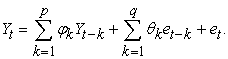(1)

Equation (1) can be written as follows: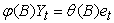(2)

where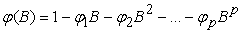and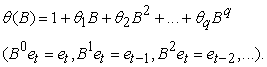If Xt is a stationary series which is obtained by d differencing the series Yt then we will have: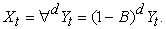(3)

Thus, the final form of the model ARIMA (p, d, q) can be expressed as follows: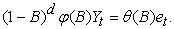(4)

A time series is called seasonal if it has at least one seasonal autoregressive parameter P (SAR) or at least one seasonal parameter of moving average Q (SMA) or both parameters (P, Q). One seasonal ARMA model is symbolized with (P, Q) where P is the number of autoregressive lag, and Q is the moving average lag and can be written as follows: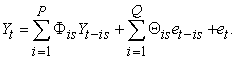(5)

One category of the univariate models is the Seasonal Autoregressive Integrated Moving Average (SARIMA) models. One seasonal ARIMA model can be expressed as SARIMA (p,d,q)(P,D,Q), where p denotes the number of autoregressive terms, q denotes the number of moving average terms and d denotes the number of times a series must be differenced to induce stationarity. P denotes the number of seasonal autoregressive components, Q denotes the number of seasonal moving average terms and D denotes the number of seasonal differences required to induce stationarity.

The previous model can be written as follows: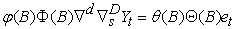(6)

where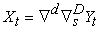is a stationary series.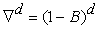represents the number of regular differences.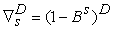represents the number of seasonal differences required to induce stationarity in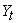.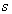is the seasonal span (=12 for monthly data).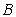is the backshift operator (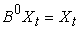,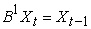,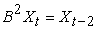, ...).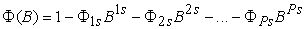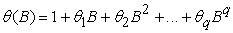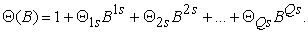3.1. Estimation of the Model SARIMA (p,d,q)(P,D,Q)

For the estimation of the model SARIMA (p,d,q)(P,D,Q), we use the Maximum Likelihood (Maximum Likelihood - ML) method, where the estimator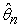of a vector of parameters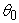can be approximated by a with meanand covariance matrix: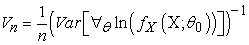(7)

where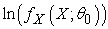is the log-likelihood of one observation from the sample, evaluated at the parameter, and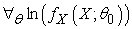is the vector of first derivatives of the log-likelihood.

For the estimate of the asymptotic covariance matrix (10), it is used the Outer Product of Gradients (OPG) estimate and it is computed as follows: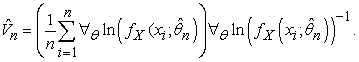(8)

Provided some regularity conditions are satisfied, the OPG estimator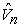is a consistent estimator of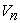. (see 19, Chapter 36).

Furthermore, for the optimization of the vectorwe use the algorithm of Broyden–Fletcher–Goldfarb–Shanno (BFGS). In , the (BFGS) algorithm is an for solving unconstrained nonlinear optimization problems and was developed by 20, 21, 22, 23. (see 18).

3.2. Diagnostic Checking of the Model SARIMA(p,d,q)(P,D,Q)

One of the statistical tools that it is used if a series has autocorrelation or heteroskedasticity is the statistic Q of 24 which is given by the formula: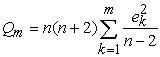(9)

where: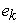is the residual autocorrelation at lag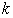,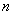is the number of residuals,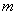is the number of time lags includes in the test. The model is considered adequate only if the p value associated with the Ljung-Box Q Statistic is higher than a given significance. For examining autocorrelation of the conditional heteroskedasticity, the correlogram of the squares of the residuals is used. If there is autocorrelation with conditional heteroskedasticity, then we apply the SARIMA-GARCH method.

3.3. The Method of SARIMA-GARCH

The method SARIMA-GARCH combines the method GARCH with the SARIMA model. The GARCH models consist of two equations, the mean equation and the variance equation. These two equations have the form: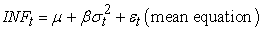(10)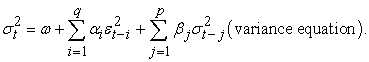(11)

We assume that for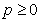and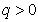, the parameters are unknown and since the variance is a positive number there must be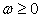and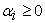for i=1,…,q and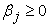for j=1,…,p.

The major disadvantage of GARCH models is their inappropriateness on the cases where we have asymmetric impact. One of the most well-known asymmetric models is the exponential model GARCH (EGARCH), and the asymmetric model GJR. The model EGARCH was suggested by 25 and is given by the formula: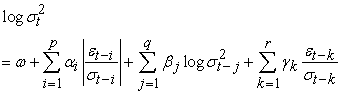(12)

where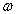,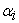,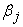and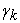are parameters that can be estimated using the maximum likelihood method.

The model GARCH-GJR(p,q) is another asymmetric GARCH model, which was suggested 26. The generalized form of the model GARCH-GJR(p,q) is given by: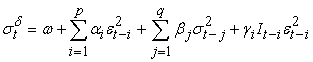(13)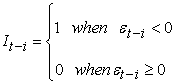where,,and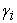are parameters that need to be estimated.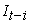is a dummy variable, meaning thatis a functional indicator that is equal to zero when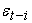is positive and it is equal to one whenis negative.

3.4. Procedure for SARIMA Modeling

• We check diagrammatically the graph of the data for the existence of seasonal variations and the possible trend.

• We observe the correlogram of the data. The coefficients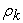may show slow or fast decrease in an exponential or wavy rate.

• If for some lag k=s the corresponding coefficient is too strong regarding to the neighboring ones, then we consider the model to have seasonality s. We isolate the autocorrelation coefficientsfor k = s, 2s, 3s and if they decrease fast then we get the seasonal differences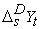in order to calculate number D of the seasonal model ARIMA(P,D,Q)s that fits to the data.

• If the existence of trend is obvious we also calculate the differences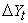for the observationsuntil stationarity is achieved. If autocorrelation is strong in the initial data, then the seasonal correlations are becoming significant in the autocorrelation diagrams after the calculation of the first differences or generally the differences of order d.

• After calculating the necessary differences (seasonal and not), we examine the new autocorrelation and partial autocorrelation diagrams of the data of the differences to identify the orders p,q and P,Q of the model ARIMA(p,d,q)(P,D,Q)s .

• To make the calculations easier we can isolate the autocorrelation coefficients with the seasonal lags s, 2s, 3s… in order to define the values of P and Q of the seasonal ARIMA(P,D,Q)s.

A seasonal model SARIMA is symbolized as SARIMA (P, D, Q) where P is the number of autoregressive lag, D is the differencing lag and Q is the moving average lag. The model can be written as follows: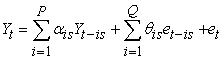(14)

### 4. Data and Empirical Results

The variable that is used in our paper is the percentage of inflation and covers the period from January of 1957 until December of 2016, comprising seven hundred and thirty two (732) monthly observations. The data were obtained from the World Bank database.

To build an ARIMA model, one essentially uses 16, which is an iterative process and involves four stages; Identification, Estimation, Diagnostic Checking and forecasting.

To evaluate the forecasting performance of various models, three different criteria have been used: the Root Mean Squared Error (RMSE), the Mean Absolute Error (MAE) and the Theil Inequality Coefficient (TIC).

In the beginning we examine the graphs of the data in the variable levels, in the first differences, and also in the seasonal differences. The graphical examination of the data is important because it can indicate if there is any structural break in the data or any outliers or any data errors. In addition, we can observe whether there is a significant seasonal pattern in the time series.

4.1. Testing for Non-stationarity

Figure 1 and Figure 2 show the plot of Greece’s monthly inflation rate and the trend analysis plot respectively.

• Figure 1. Time series plot of greece monthly inflation rate
• Figure 2. Trend plot analysis of greece monthly inflation rate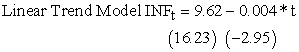From Figure 1 we observe that the initial data show the changing variance, whereas in Figure 2 the trend analysis indicates that a decreasing trend exists, which can be obvious from the significance of the trend coefficient. Therefore, we can say that the series is not stationary in its levels. Then, we calculate the first differences of the series and we examine the stationarity. Figure 3 and Figure 4 show the monthly percentages of inflation and trend analysis respectively for the first series differences.

• Figure 3. Time series plot of first difference of the original data
• Figure 4. Trend analysis for first difference of the original data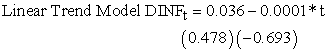From Figure 3 and Figure 4 we observe that the stationarity has been achieved since there is no trend (the coefficient in the function of linear trend is not statistically important). In order to verify the existence of stationarity in the first difference of the series, we use the unit root tests of 27, 28 and 29.

The results of Augmented Dickey–Fuller (ADF) test and Phillips-Perrons (PP) test on inflation rate series are presented in Table 1.

The results in Table 1 indicate that the inflation rate is stationary in the first differences. Therefore for our model ARIMA (p,d,q) we will have the value d=1.

Another way to examine the data of the time series is using the correlogram plots. The correlogram plot helps us define the stationarity of the series, which is important for the models of Box-Jenkins. Furthermore, we can define parameters p and q of the process ARMA, and also the seasonal parameters P and Q if they exist.

• Figure 5. Autocorrelation and partial function of first difference of the original data

In Figure 5 we observe that the coefficients in the autocorrelation function show a quick drop that verifies that the series is stationary in the first differences. In addition, since the coefficients of the autocorrelation and the partial autocorrelation functions with lags k =12 and k =24 are strong in relation to the neighboring ones, we consider that the model has seasonality s=12.

4.2. Identification of the Model

After identifying the stationarity and seasonality of the series, we define the form of the model SARIMA (p,d,q)(P,D,Q)12 from the correlogram of diagram 5. Parameters p and q can be defined from the autocorrelation and partial autocorrelation coefficients respectively by comparing them with the critical value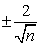. The limits for both functions (ACF, PACF) are S. From the column of autocorrelation in Figure 5 we can notice that only the value of the coefficient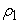(autocorrelation coefficient) is greater than the value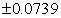, while from the column of the coefficients of partial autocorrelation the values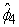(partial autocorrelation coefficient) are greater than the value. Therefore, the value of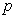will be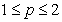,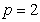and the value ofwill be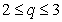respectively. The seasonal parameters from the previous diagram are defined as follows: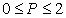and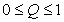. Thereafter we create Table 2 with the values ofand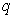and P and Q as follows.

The results from Table 2 indicate that according to the Akaike (AIC), Schwartz (SIC) and Hannan-Quinn (HQ) criteria, the SARΙMA (2,1,2)(0,1,1)12 model is the most appropriate. We move on to the next stage which is the Box-Jenkins approach that estimates the models.

4.3. Estimation of the Model

Next, we estimate the above model. Table 3 shows the results of this model.

The results of Table 3 show that the coefficients are statistically significant, so the model can be used for forecasts.

4.4. Diagnostic Checking of the SARIMA(2,1,2)(0,1,1)12 Model

In Figure 6 we check the existence of the conditional heteroskedasticity (process ARCH(q)), of the squares of the residuals of the above model.

• Figure 6. Diagnostic checking for the conditional autocorrelation of the residuals of the model SARIMA(2,1,2)(0,1,1)12

From the results of diagram 6 we observe that the autocorrelation and partial autocorrelation coefficients are statistically significant. Therefore, the null hypothesis for the absence of ARCH or GARCH model is rejected.

4.5. Estimation of the Family of GARCH Models

The estimation of the GARCH models is done with the use of maximum likelihood method. The estimations of the parameters in the logarithmic function of maximum likelihood are being calculated via nonlinear least squares using 32, and by using all the theoretic distributions. The parameters of the estimated models and the examination of the residuals of stationarity of the autocorrelation and the conditional heteroskedasticity are given in Table 4. The maximum value of the logarithm of the likelihood method (LL) gives us the best estimation.

From the previous table we observe that for all distributions the coefficients are statistically important only for the SARIMA(2,1,2)(0,1,1)12-ARCH(1) model. In this model, there is also no problem in autocorrelation and in conditional heteroskedasticity. Moreover, model SARIMA(2,1,2)(0,1,1)12-ARCH(1) has the maximum value for the logarithmic likelihood with the general error distribution (GED). Thus, we can use this model for forecasting.

Next, we estimate the following asymmetric (nonlinear) models GARCH, like the model SARIMA(2,1,2)(0,1,1)12-EGARCH(1,1) and the model SARIMA(2,1,2)(0,1,1)12-GJR-GARCH(1,1) with all the theoretic distributions. The parameters of the estimated models and the examination of the residuals of the stationarity, the autocorrelation and the conditional heteroskedasticity are given in Table 5.

From the results of Table 5 we observe that only the coefficients of the model SARIMA(2,1,2)(0,1,1)12-EGARCH(1,1) are statistically significant. Moreover, the diagnostic checks of this model do not show any problem. From the above table, we also observe that the model SARIMA(2,1,2)(0,1,1)12-EGARCH(1,1) has the maximum value for the logarithmic likelihood with the general error distribution (GED). Thus, we can use this model for forecasting.

4.6. Forecasting

The inflation forecast is done using the seasonal model SARIMA(2,1,2)(0,1,1)12 which is incorporated from the model EGARCH(1,1). For forecasting inflation, we use the stationary one-step ahead forecast, which is more accurate than the dynamic one. The stationary forecast extends the forward recursion from the end of the model forecast, allowing forecast of both the structural model and the innovations.

Having selected the form of the model SARIMA(2,1,2)(0,1,1)12-EGARCH(1,1) the graphs of the actual and predicted values of the model and of the innovations are presented in Figure 7. However, in order to see the forecast ability of the model we present some statistical indices such as the Root Mean Squared Error, the Mean Absolute Error and the Theil Inequality Coefficient in Table 6.

• Figure 7. The plot of actual values and forecast values bySARIMA(2,1,2)(0,1,1)12 -EGARCH(1,1)

From Figure 7, we conclude that the trend of forecast values follows the actual values closely.

The results of Table 6 show that MAE (Mean Absolute Error) indicates that the average difference between the forecast and the observed value of the model is 0.575, whereas RMSE (Root Mean Squared Error) and Theil Inequality Coefficient are 0.839 and 0.535 respectively. In addition, in Table 6 the forecasts for the mean and error variance of the inflation are presented with the use of diagrams. The diagram of forecast of the error variance shows that the values of inflation are evident for the time periods 1974-1975, 1979-1980 and 1990-1991. For all other periods, the diagram shows low forecast values for the error variance. This is also evident in the diagram of forecast of the mean in a wide confidence interval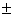2SE.

### 5. Conclusion

It is generally accepted that the major role of all central banks is to keep the inflation rates low and stable. Public and private institutions follow closely the market prices, to make decisions that allow them to optimize the use of their resources. In this context, it is very important to find a model that will predict correctly the inflation rate. The model SARIMA(2,1,2)(0,1,1)12-EGARCH(1,1) of this paper is proved to be the most suitable to forecast the inflation of the country that is examined. The model that was developed showed clearly that the estimated inflation was an important factor of the real inflation during the period of the estimation. These findings verify the fact that inflation rates of the past help us forecast future inflation.

Many researchers have developed different aspects concerning the causes of inflation, both in theoretical and empirical level. As a result, they suggest various solutions in order to face the problem. Even today the discussions made for these causes are the ones expressed by Monetarists and Structuralists. Monetarists claim that inflation would not be created if it weren’t for the money supply when it exceeds production growth. More specifically, Friedman claims that “inflation” is really a monetary phenomenon and if money supply is increasing faster than the rate of national income, then inflation is unavoidable. Thus, inflation can be limited only with the deceleration of money supply.

On the other hand, there is the Structuralists’ theory of inflation also known as “structural inflation” which explains inflation in a different manner. Structuralists support the view that increase in investment expenditure as well as money supply expansion for financing, are responsible for inflation mainly in developing countries.

The two views about inflation stated above, seem to prevail in Greece during the examined period. The great inflation increase after 1974(fall of the Greek dictatorship), justifies the Monetarists’ view of the great money supply just as in the period after 1981 with the printing of new money and the European funds flowing into the country. It can be said that during the period 1988-1992 the Structuralists’ view prevails. The following years inflation in Greece has two phases: the first is determined by the Maastricht criteria according to which inflation must be stable (no higher than 1,5 units compared to the average inflation of the three countries with the best yield) in order for a country to enter the monetary union. The second phase is the one of Troika, since 2010, during which the money supply and investments are drastically limited and consequently inflation is in low levels.

Taking into consideration the above mentioned economic periods, the present paper tries to find the appropriate model in order to forecast inflation in Greece for the following years. The model which was developed is considered the most appropriate for all the phases of inflation in this country.

### References

  Barro, J.R., Grilli, V. 2007. “European Macroeconomics”, Macmillan Education, U.K. 2007. In article View Article  Makin, J.H 2010. “Bernanke Battles U.S. Deflation Threat”, AEI. Economic Outlook, November 2010. In article  Mankiw, N. G. 2002. “Macroeconomics”. (5th ed.). Worth. Chapter. 4, 81-107. In article  Stiglitz, J. and Greenwald, B. 2003. “Towards a new paradigm in monetary economics”, Cambridge: Cambridge University Press, 2003. In article View Article  Friedman, M., Schwartz, A.J. 1970. “Monetary statistics in the U.S. estimates, sources, method”. New York: National Bureau of Economic Research (NBER) 1970. In article  Friedman, B.M., Kuttner, K.N. 1993. “Another look at the evidence on money-income causality”. Journal of Econometrics, 57, 189-203. In article View Article  Sunkel, O. 1960. “Inflation in Chile: An unorthodox approach”. International Economic Papers, 10, 107-131. In article  Olivera, J.H.G. 1964. “On structural inflation and Latin American structuralism”. Oxford Economic Papers, 16. 321-332. In article View Article  Fama, E. F. 1975. “Short-term interest rates as predictors of inflation”. American Economic Review, 65 (3), 269-268. In article View Article  Fama, E. F., Gibbons, M. R. 1982. “Inflation, real returns and capital investment”. Journal of Monetary Economics 9 (3), 297-323. In article View Article  Stockton, D.J. and Glassman, J.E 1987. “An evaluation of the forecast performance of alternative models of inflation”, The Review of Economics and Statistics, 69(1), 108-117. In article View Article  Meyler, A, K. Geoff, Quinn, T. 1998. “Forecasting Irish inflation using ARIMA models”. Central Bank and Financial Services Authority of Ireland Technical Paper Series 3/RT/98, 1-48. In article View Article  Salam, M. A., Salam, S., Feridun, M. 2007. “Modeling and forecasting Pakistan’s inflation by using time series ARIMA models”. Economic Analysis Working Papers 6, 1-10. In article View Article  Okafor, C., Shaibu, I. 2013. “Application of ARIMA models to Nigerian inflation dynamics”, Research Journal of Finance and Accounting, 4(3), 138-150. In article View Article  Cwilingiyimana, C. Mungatu, J., Harerimana, J. 2016. “Forecasting inflation in Kenya using ARIMA-GARCH Models”, International Journal of Management and Commerce Innovations, 3(2), 15-27. In article View Article  Box, G. E. P., Jenkins, G. M. 1976. “Time series analysis. Forecasting and control”. Holden-Day, San Francisco, 1976. In article View Article  Dritsaki, C. 2015. “Box–Jenkins modeling of Greek stock prices data”, International Journal of Economics and Financial Issues, 5(3), 740-747. In article View Article  Dritsaki, C. 2016. “Forecast of SARIMA Models: Αn application to unemployment rates of Greece”, American Journal of Applied Mathematics and Statistics, 4(5), 136-148. In article  Newey, W. K., McFadden, D. 1994. “Chapter 36: Large sample estimation and hypothesis testing”, Vol.4, pp. 2111-2245, in Handbook of Econometrics, Elsevier. In article PubMed  Broyden, C. G. 1970. “The convergence of a class of double-rank minimization algorithms”. Journal of the Institute of Mathematics and Its Applications. 6, 76-90. In article View Article  Fletcher, R. 1970. “A new approach to variable metric algorithms”. Computer Journal. 13(3), 317-322. In article View Article  Goldfarb, D. 1970. “A family of variable metric updates derived by variational means”. Mathematics of Computation. 24(109), 23-26. In article View Article  Shanno, D.F. 1970. “Conditioning of quasi-Newton methods for function minimization”, Mathematics of Computation. 24(111), 647-656. In article View Article  Ljung G.M., Box.G.E.P. 1978. “On a measure of a lack of fit in time series models”, Biometrika, 65(2), 297-303. In article View Article  Nelson, D.B. 1991. “Conditional heteroskedasticity in asset returns: A new approach”, Econometrica, 59, 347-370. In article View Article  Glosten, L.R., Jagannathan, R., Runkle, D.E. 1993. “On the relation between the expected value and the volatility of the nominal excess return on stocks”, The Journal of Finance, 48(5), 1779-1801. In article View Article  Dickey, DA., Fuller, WA. 1979. “Distributions of the estimators for autoregressive time series with a unit root”. Journal of American Statistical Association, 74(366), 427- 431. In article View Article  Dickey, DA., Fuller, WA. 1981. “Likelihood ratio statistics for autoregressive time series with a unit root”. Econometrica, 49(4), 1057-1072. In article View Article  Phillips, P.C.B., Perron, P. 1998. “Testing for a unit root in time series regression”. Biometrika, 75, 335-346. In article View Article  MacKinnon, J. G. 1996. “Numerical distribution functions for unit root and cointegration tests”, Journal of Applied Econometrics, 11(6), 601-618. In article View Article  Newey, W. K., West, K. D. 1994. “Automatic lag selection in covariance matrix estimation”. Review of Economic Studies, 61(4), 631-654. In article View Article  Marquardt, D.W. 1963. “An algorithm for least squares estimation of nonlinear parameters”. Journal of the Society for Industrial and Applied Mathematics, 11, 431-441. In article View ArticleThis work is licensed under a Creative Commons Attribution 4.0 International License. To view a copy of this license, visit http://creativecommons.org/licenses/by/4.0/